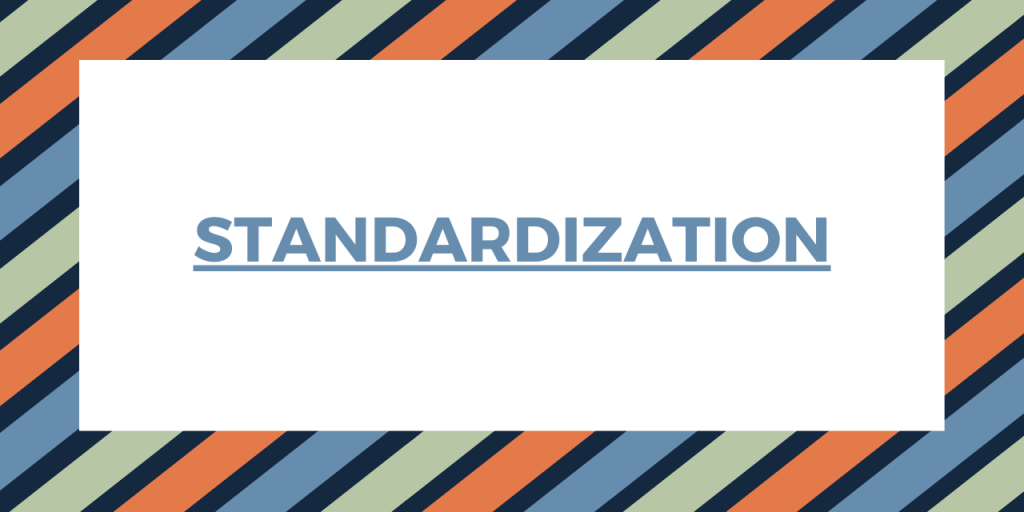# 2 Easy Ways to Standardize Data in Python for Machine LearningHey, readers. In this article, we will be focusing on 2 Important techniques to Standardize Data in Python. So, let us get started!!

## Why do we need to standardize data in Python?

Before diving deep into the concept of standardization, it is very important for us to know the need for it.

So, you see, the datasets which we use to build a model for a particular problem statement is usually built from various sources. Thus, it can be assumed that the data set contains variables/features of different scales.

In order for our machine learning or deep learning model to work well, it is very necessary for the data to have the same scale in terms of the Feature to avoid bias in the outcome.

Thus, Feature Scaling is considered an important step prior to the modeling.

Feature Scaling can be broadly classified into the below categories:

Standardization is used on the data values that are `normally distributed`. Further, by applying standardization, we tend to make the mean of the dataset as 0 and the standard deviation equivalent to 1.

That is, by standardizing the values, we get the following statistics of the data distribution

• mean = 0
• standard deviation = 1

Thus, by this the data set becomes self explanatory and easy to analyze as the mean turns down to 0 and it happens to have an unit variance.

## Ways to Standardize Data in Python

Let us now focus on the various ways of implementing Standardization in the upcoming section.

### 1. Using preprocessing.scale() function

The `preprocessing.scale(data) function` can be used to standardize the data values to a value having mean equivalent to zero and standard deviation as 1.

Here, we have loaded the IRIS dataset into the environment using the below line:

```from sklearn.datasets import load_iris
```

Further, we have saved the iris dataset to the data object as created below.

```from sklearn import preprocessing

# separate the independent and dependent variables
X_data = data.data
target = data.target

# standardization of dependent variables
standard = preprocessing.scale(X_data)
print(standard)
```

After segregating the dependent and the response/target variable, we have applied `preprocessing.scale() function` on the dependent variables to standardize the data.

Output:

### 2. Using StandardScaler() function

Python `sklearn library` offers us with `StandardScaler() function` to perform standardization on the dataset.

Here, again we have made use of Iris dataset.

Further, we have created an object of StandardScaler() and then applied `fit_transform() function` to apply standardization on the dataset.

```from sklearn.datasets import load_iris
from sklearn.preprocessing import StandardScaler

scale= StandardScaler()

# separate the independent and dependent variables
X_data = data.data
target = data.target

# standardization of dependent variables
scaled_data = scale.fit_transform(X_data)
print(scaled_data)
```

Output: